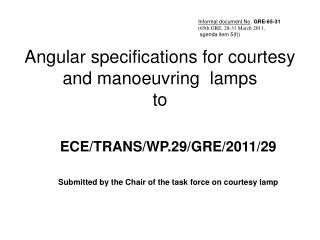DownloadDownload PresentationAngular specifications for courtesy and manoeuvring lamps to

# Angular specifications for courtesy and manoeuvring lamps to

Download Presentation## Angular specifications for courtesy and manoeuvring lamps to

- - - - - - - - - - - - - - - - - - - - - - - - - - - E N D - - - - - - - - - - - - - - - - - - - - - - - - - - -
##### Presentation Transcript

1. Angular specifications for courtesy and manoeuvring lampsto ECE/TRANS/WP.29/GRE/2011/29 Submitted by the Chair of the task force on courtesy lamp

2. There is a mistake in paragraph 6.2.1.

3. φ min= arctan ((1-mounting height)/10); with the mounting height in m φmin= arctan ((1-0.25)/10) = arctan (0.075) = 4.3° φmin= φmax+ 11,3 = 4.3 + 11.3 = 15.6° tan(15.6) = 0.279 → h = 0. 0.279 x 10000 =2790+ 250 = 3042 → ≈3m

4. φ min= arctan ((1-mounting height)/10); with the mounting height in m φmin= arctan ((1-1)/10) = arctan (0) = 0° φmin= φmax+ 11,3 = 0 + 11.3 = 11.3° tan(11.3) = 0.1998 → h = 0. 1998 x 10000 =1998+ 1000 = 3989 → ≈3m

5. φ min= arctan ((1-mounting height)/10); with the mounting height in m φmin= arctan ((1-1.5)/10) = arctan (-0.05) = -2.87° φmin= φmax+ 11,3 = -2.87 + 11.3 = 8.43° tan(8.43) = 0.1482 → h = 0. 1482 x 10000 =1482+ 1500 = 2982 → ≈3m

6. Courtesy Lamp Eye of the Observer 0° 10m Goniometer